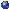The PDE theory we learn in our classes is intimately tied to the geometry of Euclidean space. We will discuss Laplace's equation and the Poisson equation on closed hypersurfaces in R^n (primarily the sphere) to illustrate some of the ways our PDE course intuition breaks down in a curved space, even with simple equations. If time permits, I will discuss the basics of my current work with my advisor which centers around a gluing construction to produce examples of the stability of Alexandrov's theorem for compact constant mean curvature hypersurfaces.